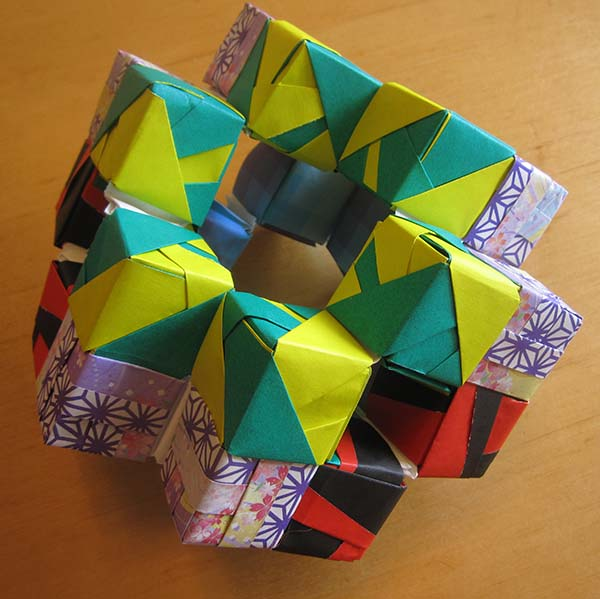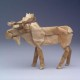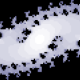# Origami: Three-formThree-Form, a model designed by me.  Some of the component cubes are taken directly from Meenakshi Mukerji.

I was looking through my photos, and I realized that there are several large models that I never got around to sharing.  This is one of them.  The Three-Form consists of 24 little cubes, assembled into a larger mathematical design.

This one is inspired by General Relativity, Einstein’s theory of gravity.  At the time I was reading Sean Carroll’s textbook on the subject, and I was lamenting how difficult it was to visualize the mathematical concepts therein.

As some of you may know, General Relativity makes use of tensors, which are generalizations of vectors.  If we have a simple vector in 4 dimensions (3 dimensions of space, and one dimension of time), we could represent the vector with its four components: vx, vy, vz, and vt.  On the other hand, a “rank-two” tensor might have 16 components: Txx, Txy, Txz, Txt, Tyx, Tyy, Tyz, Tyt, Tzx, Tzy, Tzz, Tzt, Ttx, Tty, Ttz, Ttt.  My model represents a kind of rank-three tensor, which has 64 components.

More specifically, I was trying to represent a “fully antisymmetric” tensor.  If you swap any two of the letters, you get a negative sign.  For instance, Txyz= -Txzy.  By swapping around letters, we can prove that most of the components of the tensor are zero (e.g. Txxy= 0).  What we have left are 24 components that are not zero, and each of these components is represented with a little cube.

Even though there are 24 non-zero components, most of those components are identical to each other (perhaps with a negative sign attached).  There are only four independent components, each of which is represented with a different color scheme and design.

Two explain it with less math, basically I have a 4x4x4 cube, where I’ve removed all the diagonals.  As a result, if you look at the model from any of its three main axes (x, y, or z), you see gaps along its diagonal.All in all, there are 126 separate pieces of paper.

1.bugfolder says
2.says